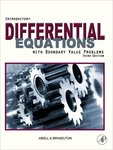## Mathematical Sciences Faculty Bookshelf#### Title

Introductory Differential Equations with Boundary Value Problems

#### Description

Georgia Southern University faculty member Martha L. Abel and James P. Braselton authored Introductory Differential Equations with Boundary Value Problems.

Book Summary: This text is for courses that are typically called (Introductory) Differential Equations, (Introductory) Partial Differential Equations, Applied Mathematics, Fourier Series and Boundary Value Problems. The text is appropriate for two semester courses: the first typically emphasizes ordinary differential equations and their applications while the second emphasizes special techniques (like Laplace transforms) and partial differential equations. The texts follows a traditional curriculum and takes the traditional (rather than dynamical systems) approach.

Introductory Differential Equations is a text that follows a traditional approach and is appropriate for a first course in ordinary differential equations (including Laplace transforms) and a second course in Fourier series and boundary value problems. Note that some schools might prefer to move the Laplace transform material to the second course, which is why we have placed the chapter on Laplace transforms in its location in the text. Ancillaries like Differential Equations with Mathematica and/or Differential Equations with Maple would be recommended and/or required ancillaries depending on the school, course, or instructor.

1-1-2009

Academic Press

#### ISBN for this edition (13-digit)

978-0123749352COinS